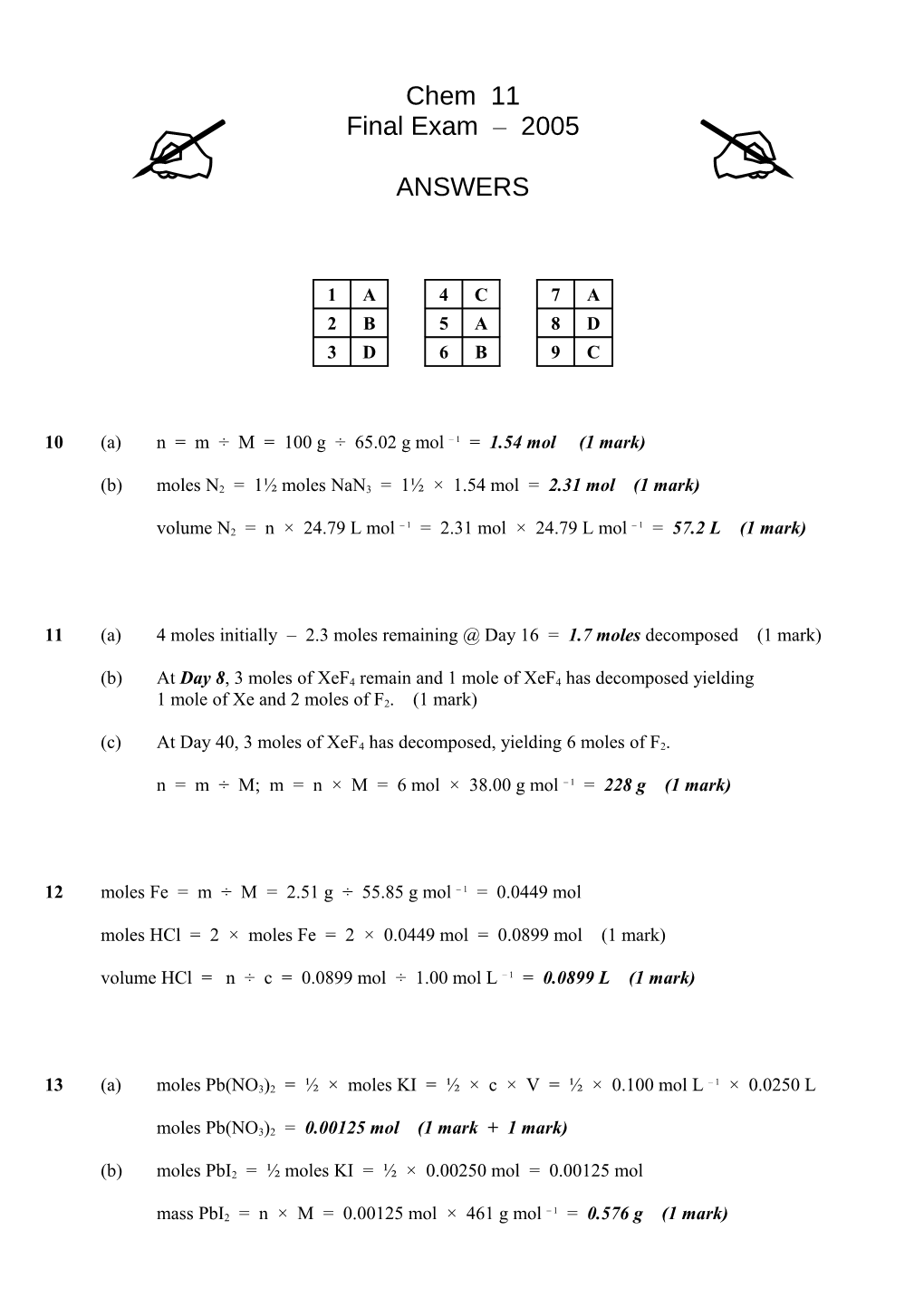# B)Moles N2 = 1 Moles Nan3 = 1 1.54 Mol = 2.31 Mol (1 Mark / Chem 11
Final Exam – 2005
1 / A / 4 / C / 7 / A
2 / B / 5 / A / 8 / D
3 / D / 6 / B / 9 / C

10(a)n = m ÷ M = 100 g ÷ 65.02 g mol – 1 = 1.54 mol (1 mark)

(b)moles N2 = 1½ moles NaN3 = 1½ × 1.54 mol = 2.31 mol (1 mark)

volume N2 = n × 24.79 L mol – 1 = 2.31 mol × 24.79 L mol – 1 = 57.2 L (1 mark)

11(a)4 moles initially – 2.3 moles remaining @ Day 16 = 1.7 moles decomposed (1 mark)

(b)At Day 8, 3 moles of XeF4 remain and 1 mole of XeF4 has decomposed yielding
1 mole of Xe and 2 moles of F2. (1 mark)

(c)At Day 40, 3 moles of XeF4 has decomposed, yielding 6 moles of F2.

n = m ÷ M; m = n × M = 6 mol × 38.00 g mol – 1 = 228 g (1 mark)

12moles Fe = m ÷ M = 2.51 g ÷ 55.85 g mol – 1 = 0.0449 mol

moles HCl = 2 × moles Fe = 2 × 0.0449 mol = 0.0899 mol (1 mark)

volume HCl = n ÷ c = 0.0899 mol ÷ 1.00 mol L – 1 = 0.0899 L (1 mark)

13(a)moles Pb(NO3)2 = ½ × moles KI = ½ × c × V = ½ × 0.100 mol L – 1 × 0.0250 L

moles Pb(NO3)2 = 0.00125 mol (1 mark + 1 mark)

(b)moles PbI2 = ½ moles KI = ½ × 0.00250 mol = 0.00125 mol

mass PbI2 = n × M = 0.00125 mol × 461 g mol – 1 = 0.576 g (1 mark)

14Sodium thiosulfate was added to hydrochloric acid in a conical flask at various temperatures.

The chemicals react to form a precipitate. The time was recorded for how long it took for the

precipitate to obscure a cross drawn on the bottom of the conical flask. As the flask was heated,

the time taken for the precipitate to obscure the cross became shorter.

Detailed description and observed results.3 marks

Detailed description of valid experiment.2 marks

Simple description of valid experiment.1 mark

15(a)Carbon or carbon monoxide or carbon dioxide (1 mark)

(b)e.g. C4H10(g) + 7O2(g) → C (s)+ CO (g)+ 2CO2(g)+ 5H2O(l) (1 mark)

► No states required.

16 indicates hydrogen bonding

Hydrogen bonding shown using dashes or any suitable representation (but not solid lines)
between correct atoms. (1 mark)

Written label indicating the hydrogen bond location. (1 mark)

17

1. Calculate the mass of strontium chloride required to be weighed.

Mass of strontium chloride = 0.250 L x 0.102 mol L-1 x [87.62 x 2(35.45)] g mol-1 = 4.04 g

1. Weigh the required quantity and dissolve in the minimum amount of water.
2. Quantitatively transfer the solution to a 250 mL volumetric flask and add enough water until the lower meniscus is just touching the fill line.
3. With the stopper on and held firmly in place, invert the volumetric flask several times to mix
the solution.

Steps 1 and 2 are credited with 1 mark. Numbers 3 and 4 are 1 mark each.

18(a)mass of iodine = 0.02 × 250 = 5 g (1 mark)

(b)mass of ethanol = mass of solution – mass of solute = 245 g (1 mark)

19(a)Density = mass H2O ÷ volume H2O = (230.5 g – 150.5 g) ÷ 80.3 mL = 0.996 g mL – 1

(b)At –10°C, water freezes to a structure where each molecule hydrogen bonds with four other molecules (tetrahedrally configured) creating a regular open structure which occupies more space, hence the greater volume and lower density. (1 mark)

(c)Ice forms on the surface of a lake (etc.) instead of the bottom allowing life to exist under
the ice during the winter.

OR

Ice is an agent of physical weathering for rocks. Successive freezing and thawing can crack open the surface of hard rock forming a component of soil. (1 mark)